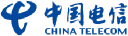# 14 High Yield Stocks With Low Debt Ratios That Are Still Cheap In Terms Of Growth

+30.92%
Upside
26.45
Market
34.63
TrefisCHA
China Telecom

This article was submitted by Dividend Yield using our Trefis Contributors tool

Stocks With Low PEG Ratios And Low Debt To Equity Researched By Dividend Yield – Stock, Capital, Investment. Sometimes, people only watch at the single P/E ratio which measures the price valuation of a company in relation to its earnings. A high P/E leads similar to a “not buy” decision. But high P/E ratios also express the growth of the company. A stock that doubles earnings every three years is it worth to pay 20 times of earnings. The price-earnings to growth (PEG) ratio is a figure that solves this problem. However, I’ve tried so screen the market by stocks that look cheap in terms of growth (a PEG ratio below one). In addition, the stocks should have a low debt to equity ratio (ratio below 0.3) and a dividend yield of more than five percent (high yields). Fourteen stocks fulfilled these criteria of which six have a double digit yield. Ten stocks have a buy or better recommendation.

Here are my favorite stocks: 1. STMicroelectronics (STM) has a market capitalization of \$6.34 billion. The company employs 53,300 people, generates revenues of \$9,735 million and has a net income of \$155.00 million. The firm’s earnings before interest, taxes, depreciation and amortization (EBITDA) amounts to \$1,643.00 million. Because of these figures, the EBITDA margin is 16.88 percent (operating margin 3.74 percent and the net profit margin finally 1.59 percent).

Relevant Articles

The total debt representing 12.95 percent of the company’s assets and the total debt in relation to the equity amounts to 20.60 percent. Due to the financial situation, a return on equity of 8.56 percent was realized. Twelve trailing months earnings per share reached a value of \$0.72. Last fiscal year, the company paid \$0.40 in form of dividends to shareholders.

Here are the price ratios of the company: The P/E ratio is 9.89, P/S ratio 0.68 and P/B ratio 0.85. Dividend Yield: 5.49 percent. The beta ratio is 1.75.

2. City Telecom (CTEL) has a market capitalization of \$510.35 million. The company employs 3,080 people, generates revenues of \$216.86 million and has a net income of \$40.49 million. The firm’s earnings before interest, taxes, depreciation and amortization (EBITDA) amounts to \$75.95 million. Because of these figures, the EBITDA margin is 35.02 percent (operating margin 22.05 percent and the net profit margin finally 18.67 percent).

The total debt representing 0.05 percent of the company’s assets and the total debt in relation to the equity amounts to 0.07 percent. Due to the financial situation, a return on equity of 18.01 percent was realized. Twelve trailing months earnings per share reached a value of \$1.02. Last fiscal year, the company paid \$0.77 in form of dividends to shareholders.

Here are the price ratios of the company: The P/E ratio is 12.93, P/S ratio 2.35 and P/B ratio 2.20. Dividend Yield: 5.84 percent. The beta ratio is 1.80.

3. Banco Santander (STD) has a market capitalization of \$74.75 billion. The company employs 193,349 people, generates revenues of \$41,996.85 million and has a net income of \$10,603.15 million. The firm’s earnings before interest, taxes, depreciation and amortization (EBITDA) amounts to \$17,390.72 million. Because of these figures, the EBITDA margin is 41.41 percent (operating margin 26.10 percent and the net profit margin finally 19.02 percent).

The total debt representing 16.95 percent of the company’s assets and the total debt in relation to the equity amounts to 277.57 percent. Due to the financial situation, a return on equity of 9.30 percent was realized. Twelve trailing months earnings per share reached a value of \$1.19. Last fiscal year, the company paid \$0.84 in form of dividends to shareholders.

Here are the price ratios of the company: The P/E ratio is 7.05, P/S ratio 1.37 and P/B ratio 0.73. Dividend Yield: 10.26 percent. The beta ratio is 1.72.

Take a closer look at the full table of low leveraged high yield stocks with cheap PEG ratios. The average price to earnings ratio (P/E ratio) amounts to 15.68 and forward P/E ratio is 23.10. The dividend yield has a value of 5.05 percent. Price to book ratio is 1.12 and price to sales ratio 3.44. The operating margin amounts to 13.93 percent.